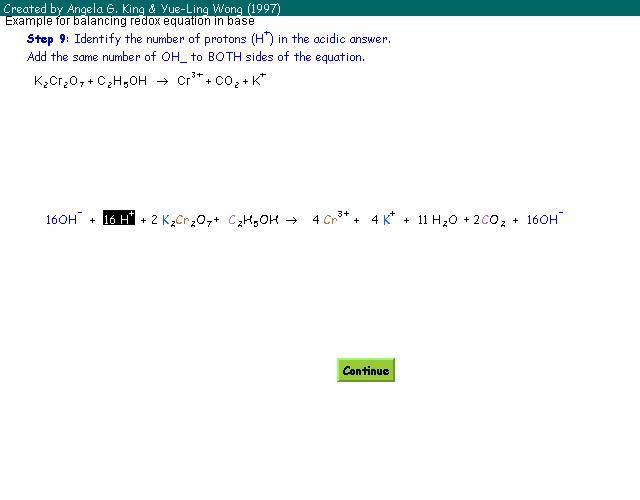Balancing Redox Equations in Base Instructor: Dr. Angela G. King (Department of Chemistry) Programming & Design: Yue-Ling Wong (Department of Computer Science and Art) Wake Forest University
The balanced equation for a redox reaction may change if the reaction is switched from an acidic solution to a basic one. The reason is fairly simple: you can't have H+ as a product or reagent in a basic solution because it would be consumed by the base!

Once you have master balancing redox reactions in acid, it is easy to do it in base. This example will balance the same reaction as we did for acidic solutions.

# Steps to Balance Redox Equations in Basic Solution

 I. Balance the equation as if in an acid solution Step 1: Separate in half reactions. Step 2: In each half reaction, balance all elements except O & H. Step 3: Balance O by adding H2O. Step 4: Balance H by adding H+. Step 5: Balance charges by adding e-. Step 6: Multiply all coefficients in 1 or both half reactions by an integer to get the number of e- in the two half reactions equal. Step 7: Add the 2 half reactions together & cancel out any species that appear on both sides of net reaction. Step 8: Check that charges and atoms are balanced. II. Balance the equation with base Step 9: Identify the number of proton (H+) in the acidic answer. Add the same number of OH- ions to BOTH sides of the equation. Step 10: If H+ and OH- appear on the same side of the equation, they will react in a 1:1 ratio to form H2O. Step 11: Cancel out water molecules that appear on both sides of the equation. Step 12: Check that charges and atoms are balanced.

# An online interactive tutorial to show you step-by-step how to apply the steps:Update 2020-11: The Adobe Shockwave is no longer supported but I have received requests to access this interactive tutorial. So now I have made this interactive tutorial available as an executable for Windows.